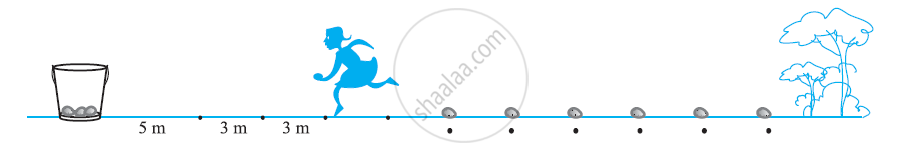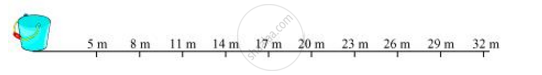# In a potato race, a bucket is placed at the starting point, which is 5 m from the first potato and other potatoes are placed 3 m apart in a straight line. There are ten potatoes in the line. - Mathematics

In a potato race, a bucket is placed at the starting point, which is 5 m from the first potato and other potatoes are placed 3 m apart in a straight line. There are ten potatoes in the line.A competitor starts from the bucket, picks up the nearest potato, runs back with it, drops it in the bucket, runs back to pick up the next potato, runs to the bucket to drop it in, and she continues in the same way until all the potatoes are in the bucket. What is the total distance the competitor has to run?

[Hint: to pick up the first potato and the second potato, the total distance (in metres) run by a competitor is 2 × 5 + 2 ×(5 + 3)]

#### SolutionThe distances of potatoes are as follows.

5, 8, 11, 14…

It can be observed that these distances are in A.P.

a = 5

d = 8 − 5 = 3

S_n = n/2[2a+(n-1)d]

S_10=10/2[2(5)+(10-1)3]

= 5[10 + 9 × 3]

= 5(10 + 27) = 5(37)

= 185

As every time she has to run back to the bucket, therefore, the total distance that the competitor has to run will be two times of it.

Therefore, total distance that the competitor will run = 2 × 185

= 370 m

Alternatively,

The distances of potatoes from the bucket are 5, 8, 11, 14…

Distance run by the competitor for collecting these potatoes are two times of the distance at which the potatoes have been kept. Therefore, distances to be run are

10, 16, 22, 28, 34,……….

a = 10

d = 16 − 10 = 6

S10 =?

S_10 = 10/2[2xx10+(10-1)6]

= 5[20 + 54]

= 5 (74)

= 370

Therefore, the competitor will run a total distance of 370 m.

Concept: Sum of First n Terms of an AP
Is there an error in this question or solution?

#### APPEARS IN

NCERT Class 10 Maths
Chapter 5 Arithmetic Progressions
Exercise 5.3 | Q 20 | Page 114